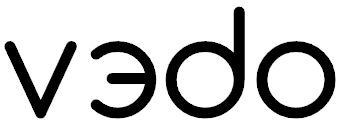Fork me on GitHub

A python module for scientific analysis and visualization of эd objects

## Features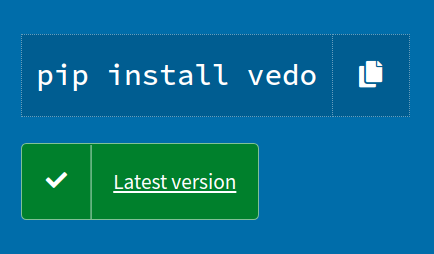## Quick Start

To visualize a file from a web URL (or from your Dropbox!), simply type in your terminal:

``````pip install vedo
vedo https://vedo.embl.es/examples/data/panther.stl.gz``````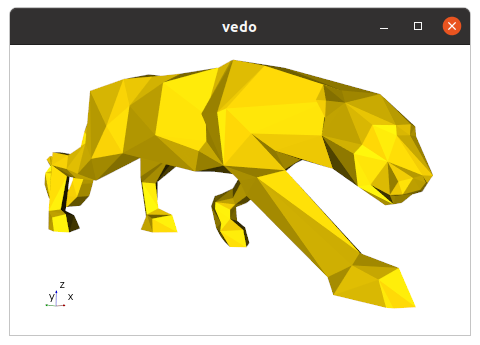To visualize a full 3D interactive scene, type:

``vedo https://vedo.embl.es/examples/geo_scene.npz``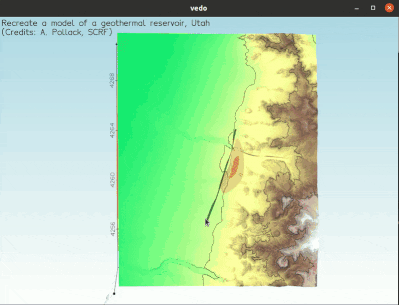Let's create an interactive 3D scene with a simple cone, in 3 lines of python code:

``````from vedo import Cone

# Create a simple cone
c = Cone()

# Show it (with axes)
c.show(axes=1)

# Now you can interact with the 3D scene,
# press "h" to explore the possibilities! ``````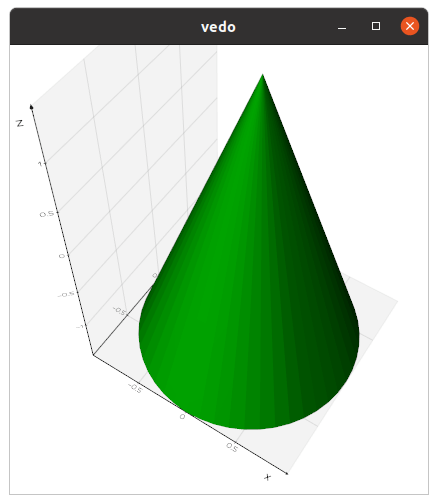Let's write a simple python script that loads a polygonal Mesh and generates a cool rendering by adding some custom light sources to the scene:

``````from vedo import *

# Load a polygonal mesh, make it white and glossy:
man = Mesh('https://vedo.embl.es/example/data/man.vtk')
man.c('white').lighting('glossy')

# Create two points:
p1 = Point([ 1,0,1], c='yellow')
p2 = Point([-1,0,2], c='red')

# Add colored light sources at the point positions:
l1 = Light(p1, c='yellow')
l2 = Light(p2, c='red')

# Show everything in one go:
show(man, l1, l2, p1, p2, "Hello World", axes=True)``````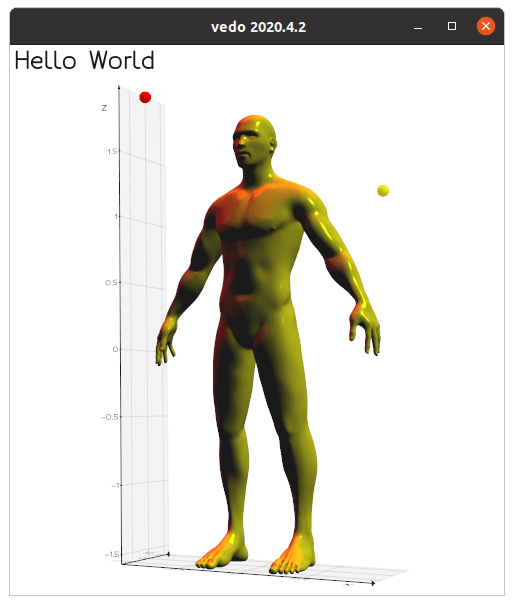Let's create a Volume - a volumetric dataset - from a numpy object:

``````import numpy as np
from vedo import *

# Create a scalar field: the distance from point (15,15,15)
X, Y, Z = np.mgrid[:30, :30, :30]
scalar_field = ((X-15)**2 + (Y-15)**2 + (Z-15)**2)/225

# Create the Volume from the numpy object
vol = Volume(scalar_field)

# Generate the surface that contains all voxels in range [1,2]

show(lego, axes=True)``````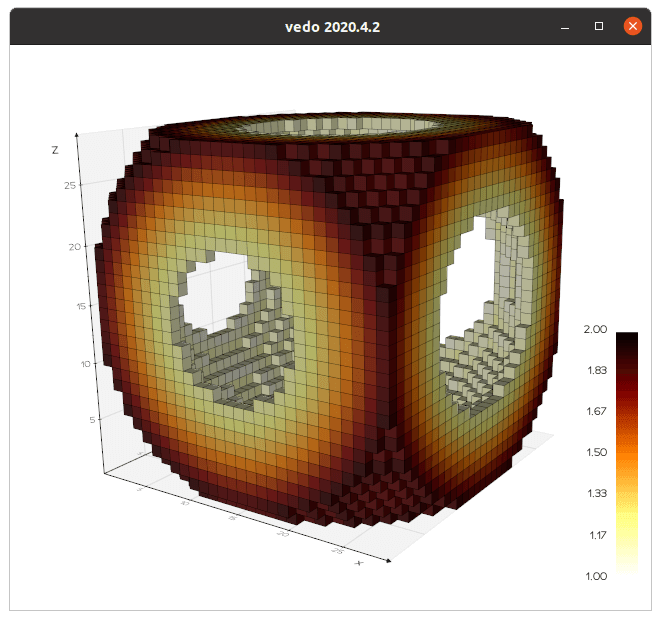Draw a creative scatter plot of a boring 2D Gaussian distribution.
Let the marker size be proportional to sin(2y) and the Red level of its [R,G,B] color proportional to sin2(2x).
Finally add a simple 1D histogram of variable x on the top of it:

``````from vedo.pyplot import plot, histogram, show
import numpy as np

n = 2000
x = np.random.randn(n)
y = np.random.randn(n)
R = np.sin(2*x)**2

marker_sizes = np.sin(2*y)/8
marker_cols  = np.c_[R, np.zeros(n), np.zeros(n)]  # RGB

p = plot(x, y,
marker=">",           # marker style
ms=marker_sizes,      # marker size array
mc=marker_cols,       # marker color array
ma=0.2,               # const. marker alpha
lw=0,                 # no line width
aspect=4/3,           # plot aspect ratio
)
h = histogram(x, aspect=18/3).shift(0, p.ybounds(1)*1.1)
show(p, h)``````### References

Scientific publications leveraging `vedo` (formerly known as `vtkplotter`):
• X. Diego et al.: "Key features of Turing systems are determined purely by network topology", Physical Review X, 20 June 2018.
• M. Musy, K. Flaherty et al.: "A Quantitative Method for Staging Mouse Limb Embryos based on Limb Morphometry", Development, 5 April 2018, DOI.
• F. Claudi, A. L. Tyson, T. Branco, "Brainrender. A python based software for visualisation of neuroanatomical and morphological data.", DOI.
• J. S. Bennett, D. Sijacki, "Resolving shocks and filaments in galaxy formation simulations: effects on gas properties and star formation in the circumgalactic medium", Monthly Notices of the Royal Astronomical Society, Volume 499, Issue 1, November 2020, DOI.
• G. Dalmasso et al., "Evolution in space and time of 3D volumetric images", in preparation.
Presentations at international conferences:
• M. Musy, G. Dalmasso, J. Sharpe and N. Sime, "`vedo`: plotting in FEniCS with python", Poster at FEniCS'2019, SDTM, Washington DC, June 2019.
• G. Dalmasso, "Evolution in space and time of 3D volumetric images". Talk at Image-based Modeling and Simulation of Morphogenesis. Max Planck Institute for the Physics of Complex Systems, Dresden, Germany, March 2019.
• G. Dalmasso, "A four-dimensional growing mouse limb bud reconstruction". Talk at SEBD, Spain, November 2020.
You can cite `vedo` as:
• M. Musy et al., "`vedo`, a python module for scientific analysis and visualization of 3D objects and point clouds", Zenodo, 2021, doi.org/10.5281/zenodo.4287635.